# Electro chemistry Notes for Class 12 Chemistry

0
1509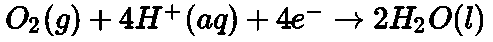CBSE Class 12 Chemistry
Quick Revision Notes
Chapter 3
Electrochemistry

• Oxidation: It is defined as a loss of electrons while reduction is defined as a gain of electrons.

In a redox reaction, both oxidation and reduction reaction takes place simultaneously.

• Direct redox reaction: In a direct redox reaction, both oxidation and reduction reactions take place in the same vessel. Chemical energy is converted to heat energy in a direct redox reaction.
• Indirect redox reaction: In indirect redox reactions, oxidation and reduction take place in different vessels.

In an indirect redox reaction, chemical energy is converted into electrical energy.The device which converts chemical energy into electrical energy is known as an electrochemical cell.

• In an electrochemical cell:
1. The half-cell in which oxidation takes place is known as oxidation half-cell
2. The half-cell in which reduction takes place is known as reduction half-cell.
3. Oxidation takes place at anode which is negatively charged and reduction takes place at cathode which is positively charged.
4. Transfer of electrons takes place from anode to cathode while electric current flows in the opposite direction.
5. An electrode is made by dipping the metal plate into the electrolytic solution of its soluble salt.
6. A salt bridge is a U shaped tube containing an inert electrolyte in agar-agar and gelatine.
• Salt bridge: A salt bridge maintains electrical neutrality and allows the flow of electric current by completing the electrical circuit.
• Representation of an electrochemical cell:
1. Anode is written on the left while the cathode is written on the right.
2. Anode represents the oxidation half-cell and is written as: Metal/Metal ion (Concentration)
3. Cathode represents the reduction half-cell and is written as: Metal ion (Concentration)/Metal
4. Salt bridge is indicated by placing double vertical lines between the anode and the cathode
5. Electrode potential is the potential difference that develops between the electrode and its electrolyte. The separation of charges at the equilibrium state results in the potential difference between the metal and the solution of its ions. It is the measure of tendency of an electrode in the half cell to lose or gain electrons.
6. Standard electrode potential: When the concentration of all the species involved in a half cell is unity, then the electrode potential is known as standard electrode potential. It is denoted as E0.
• According to the present convention, standard reduction potentials are now called standard electrode potential.
• Types of electrode potential: There are 2 types of electrode potentials namely,
1. Oxidation potential
2. Reduction potential
• Oxidation potential: It is the tendency of an electrode to lose electrons or get oxidized.
• Reduction potential: It is the tendency of an electrode to gain electrons or get reduced.

Oxidation potential is the reverse of reduction potential.

• The electrode having a higher reduction potential have higher tendency to gain electrons and so it acts as a cathode whereas the electrode having a lower reduction potential acts as an anode.
• The standard electrode potential of an electrode cannot be measured in isolation.
• According to convention, the Standard Hydrogen Electrode is taken as a reference electrode and it is assigned a zero potential at all temperatures.
• Reference electrode: Standard calomel electrode can also be used as a reference electrode
• SHE: Standard hydrogen electrode consists of a platinum wire sealed in a glass tube and carrying a platinum foil at one end. The electrode is placed in a beaker containing

an aqueous solution of an acid having 1 Molar concentration of hydrogen ions. Hydrogen gas at 1 bar pressure is continuously bubbled through the solution at 298 K. The oxidation or reduction takes place at the Platinum foil. The standard hydrogen electrode can act as both anode and cathode.

• If the standard hydrogen electrode acts as an anode: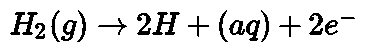If the standard hydrogen electrode acts as a cathode: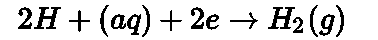In the electrochemical series, various elements are arranged as per their standard reduction potential values.

A substance with higher reduction potential value means that it has a higher tendency to get reduced. So, it acts as a good oxidising agent.

A substance with lower reduction potential value means that it has a higher tendency to get oxidised. So, it acts as a good reducing agent.

The electrode with higher reduction potential acts as a cathode while the electrode with a lower reduction potential acts as an anode.

The potential difference between the 2 electrodes of a galvanic cell is called cell potential and is measured in Volts.

The cell potential is the difference between the reduction potential of cathode and anode.

E cell = E cathode – E anode

Cell potential is called the electromotive force of the cell (EMF) when no current is drawn through the cell.

Nernst studied the variation of electrode potential of an electrode with temperature and concentration of electrolyte.

Nernst formulated a relationship between standard electrode potential E0 and electrode potential E.At equilibrium, cell potential Ecteell becomes zero.

Relationship between equilibrium constant Kc and standard cell potential E0cell: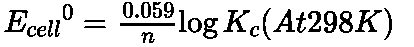Work done by an electrochemical cell is equal to the decrease in Gibbs energy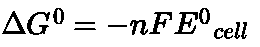The substances which allow the passage of electricity through them are known as conductors.

Every conducting material offers some obstruction to the flow of electricity which is called resistance. It is denoted by R and is measured in ohm.

The resistance of any object is directly proportional to its length l and inversely proportional to its area of cross section A.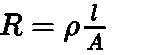Where p is called specific resistance or resistivity.

The SI unit of specific resistivity is ohm metre.

The inverse of resistance is known as conductance, G

Unit of conductance is ohm-1 or mho. It is also expressed in Siemens denoted by S. The inverse of resistivity is known as conductivity. It is represented by the symbol The SI unit of conductivity is Sm-1. But it is also expressed in Scm-1.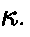Conductivity = Conductance * Cell constant

For measuring the resistance of an ionic solution, there are 2 problems:

1. Firstly, passing direct current changes the composition of the solution
2. Secondly, a solution cannot be connected to the bridge like a metallic wire or a solid conductor.

Conductivity cell: The problem of measuring the resistance of an ionic solution can be resolved by using a source of alternating current and the second problem is resolved by using a specially designed vessel called conductivity cell.

A conductivity cell consists of 2 Pt electrodes coated with Pt black. They have area of cross section A and are separated by a distance T. Resistance of such a column of solution is given by the equation:

Whereis called cell constant and is denoted by the symbol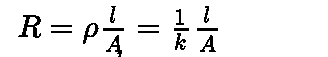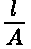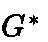Molar conductivity of a solution: It is defined as the conducting power of all the ions

/ 7

produced by dissolving 1 mole of an electrolyte in solution.

Molar conductivity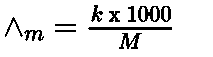WhereConductivity and M is the molarity Unit of Molar conductivity is Scm2 mol- 1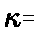• Equivalent conductivity: It is the conductivity of all the ions produced by dissolving one gram equivalent of an electrolyte in solution. Unit of equivalent conductivity is S cm2 (g equiv) -1

Equivalent conductivity: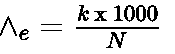• Kohlrausch’s Law of independent migration of ions: According to this law, molar conductivity of an electrolyte, at infinite dilution, can be expressed as the sum of individual contributions from its individual ions.
• If the limiting molar conductivity of the cations is denoted byand that of the anions bythen the limiting molar conductivity of electrolyte is: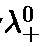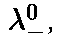Molar conductivity,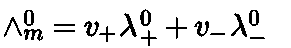Where v+ and v- are the number of cations and anions per formula of electrolyte

• Degree of dissociation: It is ratio of molar conductivity at a specific concentration ‘c’ to the molar conductivity at infinite dilution. It is denoted by.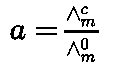• Dissociation constant:WhereKa is acid dissociation constant, ‘c’ is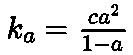concentration of electrolyte, a is degree of ionization.

• Faraday constant: It is equal to charge on 1 mol of electrons. It is equal to 96487 C mol-1 or approximately equal to 96500 C mol-1.
• Faraday’s first law of electrolysis: The amount of substance deposited during electrolysis is directly proportional to quantity of electricity passed.
• Faraday’s second law of electrolysis: If same charge is passed through different electrolytes, the mass of substance deposited will be proportional to their equivalent weights.
• Products of electrolysis: The products of electrolysis depend upon
• The nature of electrolyte being electrolyzed and the nature of electrodes. If electrode is inert like platinum or gold, they do not take part in chemical reaction i.e. they neither lose nor gain electrons. If the electrodes are reactive then they will take part in chemical reaction and products will be different as compared to inert electrodes.
• The electrode potentials of oxidizing and reducing species. Some of the

electrochemical processes although feasible but slow in their rates at lower voltage, these require extra voltage, i.e. over voltage at which these processes will take place. The products of electrolysis also differ in molten state and aqueous solution of electrolyte.

• Primary cells: A primary cell is a cell in which electrical energy is produced by the reaction occurring in the cell, e.g. Daniel cell, dry cell, mercury cell. It cannot be recharged.
• Dry Cell:

At anode At cathode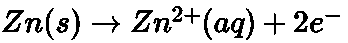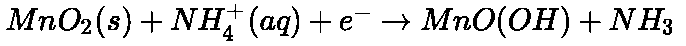The net reaction: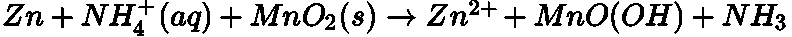• Mercury Cell: The electrolyte is a paste of KOH and ZnO.

At Anode: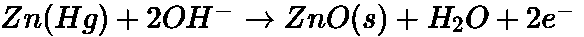At cathode: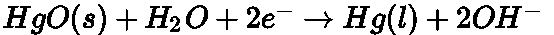The net reaction: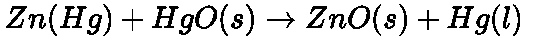• Secondary cells: Those cells which are used for storing electricity, e.g., lead storage battery, nickel – cadmium cell. They can be recharged.

Anode: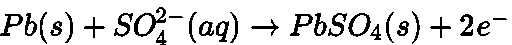Cathode: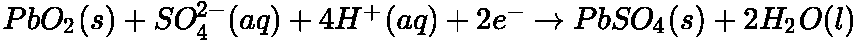The overall cell reaction consisting of cathode and anode reactions is: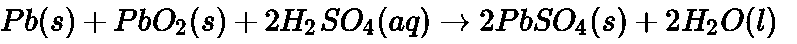On recharging the battery, the reaction is reversed.

• Nickel cadmium cell: It is another type of secondary cell which has longer life than lead storage cell but more expensive to manufacture.

The overall reaction during discharge is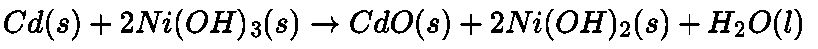• Fuel cells:

At Anode: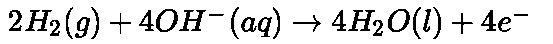At cathode: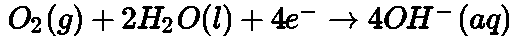• Overall reaction: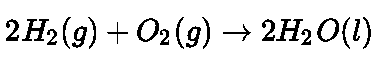• Corrosion:

Oxidation: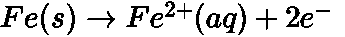Reduction:• Galvanization: It is a process of coating zinc over iron so as to protect it from rusting.
• Cathodic protection: Instead of coating more reactive metal on iron, the use of such metal is made as sacrificial anode.
1. Electrode potential increases with increase in the concentration of the electrolyte and decrease in temperature.

Nernst equation when applied to a cell, it helps in calculating the cell potential.

Previous articleSolutions Notes for Class 12 Chemistry
Next articleChemical Kinetics Notes for Class 12 Chemistry